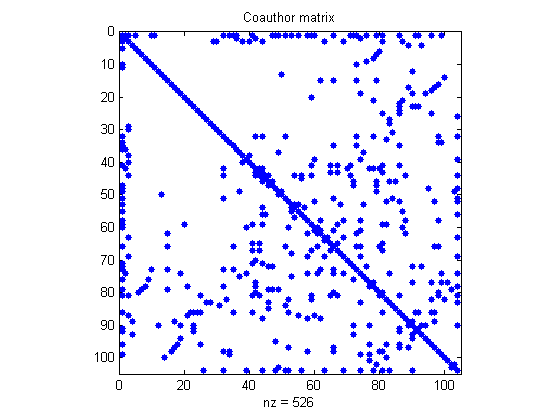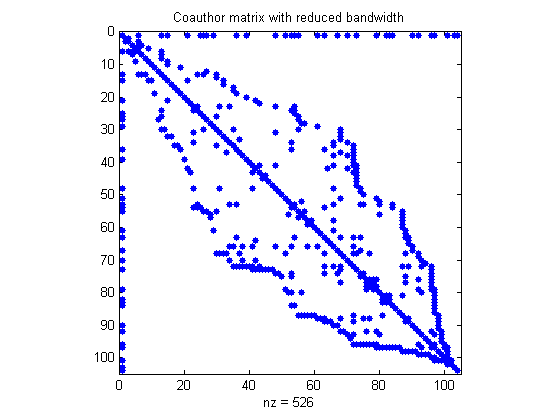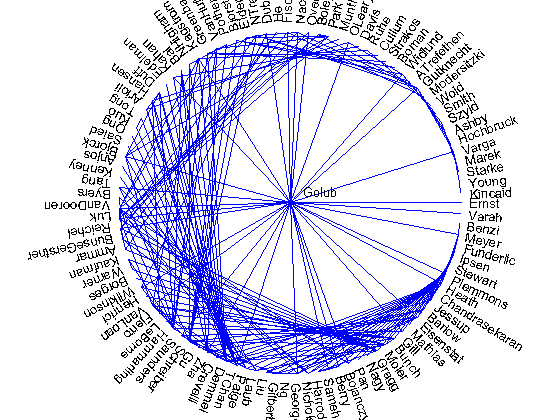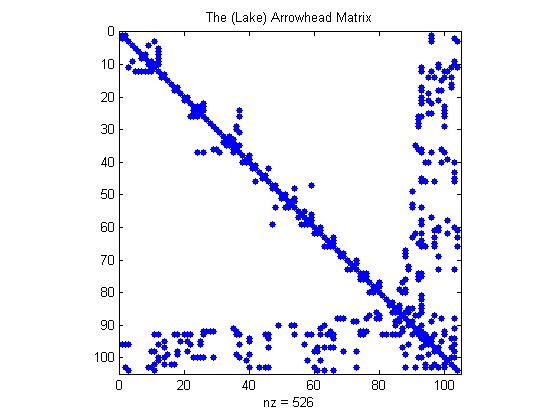# Lake Arrowhead Coauthor Graph

Twenty years ago, during the Householder Symposium at the Lake Arrowhead conference center , John Gilbert carried out one of the world's first computational social network analyses.

### Contents

#### Householder XII

Twenty years ago, in June, 1993, the UCLA Lake Arrowhead Conference Center in the San Bernardino Mountains, 90 miles east of Los Angeles, was the site of the Householder XII Symposium on Numerical Linear Algebra, organized by Gene Golub and Tony Chan.

#### John Gilbert Remembers

John Gilbert is now a Professor at U. C. Santa Barbara. A couple of years before the Lake Arrowhead meeting, in 1990 and 1991, John, Rob Schreiber, and I had developed the initial implementation of sparse matrices in MATLAB. John's research activities now include sparse matrix techniques for graph and social network problems. He recently wrote to me in an email, "It was at Householder 1993 in Lake Arrowhead that we used MATLAB's sparse matrices to do the first computational social network analysis I ever saw."

John used to be at PARC, the Xerox Palo Alto Research Center. A file containing his work from 1993 was archived at PARC, but is no longer available,(ftp://ftp.parc.xerox.com/pub/gilbert/graph.html). Most of this blog post was derived from that archived work.

#### Coauthor Matrix

Nick Trefethen posted a flip chart with Gene Golub's name in the center. He invited everyone present to add their name to the chart and draw lines connecting their name with the names of all their coauthors. The diagram grew denser throughout the week. At the end it was a graph with 104 vertices (or people) and 211 edges. We entered the names and coauthor connections into MATLAB, creating an adjacency matrix A. Let's retrieve the names and matrix as they are stored in the PARC archive.

load housegraph
size(A)

ans =
104   104


The matrix A is 104-by-104 and symmetric. Elements A(i,j) and A(j,i) are both equal to one if i and j are the indices of coauthors and equal to zero otherwise. Most of the elements are zero because most pairs of attendees are not coauthors. So the matrix is sparse. In fact, the sparsity, the fraction of nonzero elements, is less that five percent.

format short
sparsity = nnz(A)/prod(size(A))

sparsity =
0.0486


Here is a picture of the matrix, a spy plot that shows the location of the nonzeros, with Golub in the first column and the other authors in the fairly arbitrary order in which they appeared on Trefethen's flip chart.

spy(A);
title('Coauthor matrix');
snapnow#### Most Prolific

As the adjacency matrix is loaded from the archive, the most prolific coauthor is already in the first column. It was not a surprise to those of us at the conference to find that the most prolific coauthor is Gene Golub.

m = find(sum(A) == max(sum(A)))
name(m,:)

m =
1
ans =
Golub


#### Reorder the Authors

We want to create a circular plot with Golub in the center, the other authors around the circumference, and edges connecting the coauthors. If we were to place the authors around the circumference in the order we retrieve them from the chart, the edges would cross the circle pretty much randomly. We want to rearrange the authors so that the coauthor connections are as close as possible to the circumference of the circle. This corresponds to a symmetric permutation of the matrix that minimizes, or at least reduces, its bandwidth.

#### Reverse Cuthill McKee

The Reverse Cuthill McKee algorithm is the only algorithm I know that is usually used backwards. In 1969 Elizabeth Cuthill and J. McKee described a heuristic for reordering the rows and columns of a matrix to reduce its bandwidth. In 1991 J. Alan George pointed out that reversing the Cuthill McKee ordering almost always leads to fewer arithmetic operations in Gaussian elimination. The algorithm applies to symmetric matrices, so MATLAB has a symrcm function, but no symcm function. Here we are reducing bandwidth; we are not doing any arithmetic, so the difference between Cuthill-McKee and Reverse-Cuthill-McKee is purely cosmetic.

#### Coauthor Graph

r = symrcm(A(2:end,2:end));
prcm = [1 r+1];
spy(A(prcm,prcm))
title('Coauthor matrix with reduced bandwidth');Now we are were able to actually plot the coauthor graph. Fortunately, plotting text at arbitrary angles had just been provided in MATLAB.

drawit;
snapnow#### My Connectivity

You will find my name at about 5 o'clock on the coauthor graph. There is no edge connecting me to Golub because Gene and I were (not yet) coauthors. But how many coauthors do we have in common? Taking the second power of the connectivity matrix gives the paths of length two.

A2 = A^2;
count = A2(Golub,Moler)

count =
(1,1)        2


And who are those common coauthors?

twos = name( find ( A(:,Golub) .* A(:,Moler) ), : )

twos =
Wilkinson
VanLoan


Finally, we find a minimum degree reordering of the matrix and discover its true nature as, of course, ....

pmd = amd(A);
spy(A(pmd,pmd))
title('The (Lake) Arrowhead Matrix');Published with MATLAB® R2013b

|